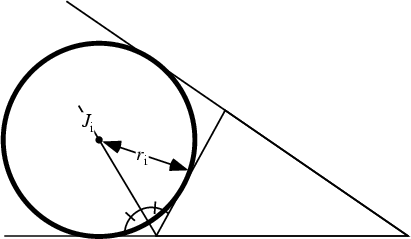The Radius of an Excircle. Let a Triangle have exradius(sometimes denoted), opposite sideand angle, Area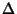, and Semiperimeter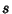. Then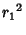(1)(2)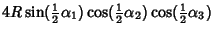(3)

(Johnson 1929, p. 189), where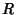is the Circumradius. Let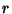be the Inradius, then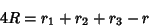(4)(5)(6)

Some fascinating Formulas due to Feuerbach are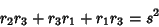(7)(8)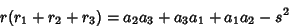(9)(10)(11)

(Johnson 1929, pp. 190-191).

References

Johnson, R. A. Modern Geometry: An Elementary Treatise on the Geometry of the Triangle and the Circle. Boston, MA: Houghton Mifflin, 1929.

Mackay, J. S. Formulas Connected with the Radii of the Incircle and Excircles of a Triangle.'' Proc. Edinburgh Math. Soc. 12, 86-105.

Mackay, J. S. Formulas Connected with the Radii of the Incircle and Excircles of a Triangle.'' Proc. Edinburgh Math. Soc. 13, 103-104.